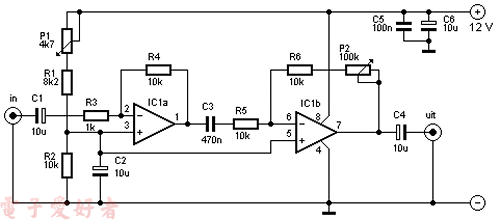# NE5532麦克风前置放大器R1 = 8,2 kΩ
R2, R4, R5, R6 = 10 kΩ
R3 = 1 kΩ
P1 = 4,7 kΩ
P2 = 100 kΩ
C1, C2, C4, C6 = 10 µF
C3 = 470 nF
C5 = 100 nF
IC1 = NE 5532